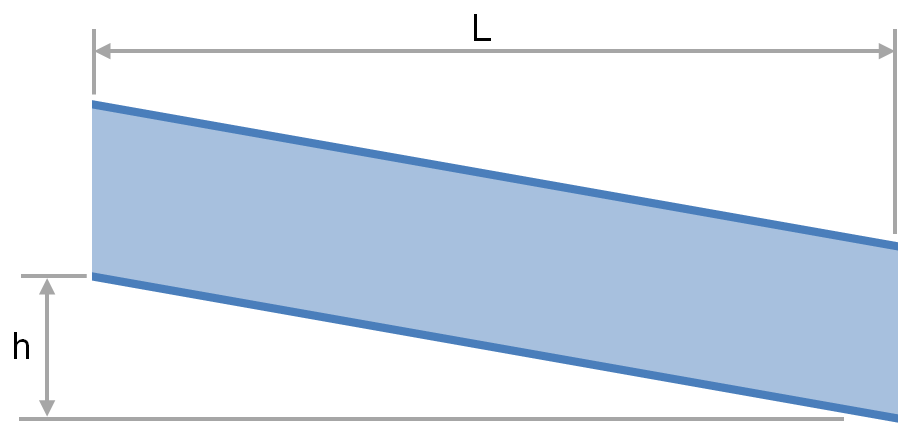# Summary

The transport of fluid under gravity is often achieved using partially filled pipes, channels, flumes, ditches and streams. To determine the slope and elevation change required or the flow rate that is achievable one must be able to calculate the head loss and friction factor. This article provides relationships for the calculation of head loss and friction factor for fluids flowing via these conduits.

# Definitions

 : Cross sectional area of flow : Chezy constant : Darcy friction factor : Gravity : Head loss dues to friction (equal to liquid surface height change over channel length) : Length of channel : Manning characteristic roughness : Wetted perimeter of channel : Volumetric Flow Rate : Hydraulic radius : Slope of the channel bottom : Friction slope of the channel : Average velocity

# Introduction

There are many applications, particularly in the water industries where liquid is transported under gravity either through open topped conduits, natural streams or through partially filled pipes. For these systems it is required to calculate parameters such as flow rate, head loss, required elevation change and the required slope of the system.

In this application we cannot use well understood formulae for fluid flow through a pipe under pressure, however using the modifications and extensions to those relationships described below we can design and analyse these systems.In this article we use Manning’s characteristic roughness to determine the friction factor, which we can then use in modified versions of the standard flow equations to determine the head, flow rate or slope of the system.

# Equations for Flow Open Channels and Partially Filled Pipes

The following table summarises the equations used to calculate the primary parameters of interest for the flow in open channels and partially filled pipes. The subsequent sections cover each of the components used in the equations below.

Propertyusing using
Friction Factor

Velocity

Volumetric Flow Rate

Slope

# Hydraulic Radius and Hydraulic Diameter

For free surface flows it is customary to use hydraulic radius as the characteristic dimension. To avoid confusion it is worth noting that the hydraulic radius is not equivalent to half of the hydraulic diameter. The hydraulic radius may be calculated as follows:

This gives the result that the hydraulic radius is 4 times the hydraulic diameter.

# Head Loss in Open Channels

The head loss for flow in an open channel can be expressed in terms similar to that for enclosed pipe, using the hydraulic radius and the length of the channel.

# The Slope of a Channel or Stream

The frictional slope of a stream is required in order to calculate the velocity of a stream in an open channel or partially filled pipe. For streams of uniform width and liquid depth, the friction slope of the channel is equal to the slope of the bottom of the channel:For streams which change in width or height the assumption that the friction slope and the slope of the bottom of the channel are equal should not be used.

# The Chezy Coefficient and Equation

Often it is desired to calculate the maximum velocity of a stream. This can be done using the equation presented below:

Where the first group in the above equation is often expressed as the Chezy coefficient .

and, the following is known as the Chezy equation:

The Chezy coefficient characterises the shape and roughness of a channel or stream into a single number, and thus is a way of comparing open flow systems. A larger Chezy coefficient corresponds to a higher flow rate, all other factors being equal.

# Manning Characteristic Roughness

Similar to the Chezy coefficient the Manning characteristic roughness characterises the resistance of a channel of stream to flow based on the shape and materials of the boundaries. Unlike the Chezy coefficient it is independent of the size of the flow path. A larger Manning characteristic roughness corresponds to a lower flow rate, all other factors being equal.

## Relationship to the Chezy Coefficient

The Manning characteristic roughness ( ) allows us to determine the value of the Chezy coefficient.

Refer to our article for typical values of the Manning characteristic roughness.

1. Water Resources Engineering2. Hydraulic Engineering3. Perry's Chemical Engineers' Handbook, Eighth Edition4. Marks' Standard Handbook for Mechanical Engineers 11th Edition